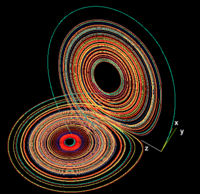Mathematics

The third, youngest and most numerous in terms of the number of students, field at the Faculty of Technical Physics and Applied Mathematics is Mathematics with Applied Mathematics, Financial Mathematics and Biomathematics as majors.The decision to choose the majors in mathematical studies is made by the student on the basis of his/her plans and interests at the end of the second year of studies. The best students in both majors are entitled to individual studies on the basis of their own program approved by the appointed academic supervisor. Students pursuing individual courses of study are practically by no means restricted in choosing their subjects as long as the curriculum minimum prescribed for master studies in mathematics is observed. Studies in the field of Mathematics at Gdańsk University of Technology are essentially different from analogous university studies. The basic difference is focusing on the application of mathematics in the broad sense. The curriculum of those studies includes many technical subjects hence a graduate from our university receives not only a master's degree as is the case with non-technical universities but a master's and an engineer's degree. Owing to that our graduate will have a diploma of Master of Science, Engineer in Mathematics, with gives much more scope in employment prospects.

During mathematical studies knowledge from the basic fields of mathematics such as analysis and algebra is acquired, and then, more specialist fields are pursued including:

• theory of probability;
• numerical methods;
• differential equations;
• functional analysis;
• topology;
• optimisation and programming methods;
• introduction to information science and discrete mathematics;
• symbolic calculations;
• computer software development methods;
• statistics;
• financial mathematics.The first semesters of studies in the field of Mathematics focus mainly on the basics of higher mathematics and information technology. Students learn the basics of mathematical analysis, algebra and theory of graphs. Then, the program covers subjects related to differential equations, functional analysis and applications of mathematics using computers and learning programming languages. Great emphasis is laid on developing practical skills of independent conceptual work - in analysis and specification of computer programs and developing algorithms - as well as practical work - software writing and testing. In final semesters a large number and variety of specialist lectures allow students to familiarize themselves with specific technical problems and methods how to solve them. They comprise mathematical modelling (including thermal conduction and diffusion phenomena modelling), non-linear computational mechanics, numerical methods of algebra and differential equations, control theory, operations research, dynamic systems in technology, cryptography, chaos theory, game theory and financial mathematics.

A graduate from Mathematics having Applied Mathematics as the major will have a master's degree and an engineer's degree and wide background in mathematics with a special skill to use approximate methods. He/She will be prepared to solve, in cooperation with other specialists, difficult technical problems based on a good mathematical and IT background.

Graduates from that field will be able to find employment as specialists in mathematics at universities of technology, scientific research institutes, computational centres, banks, statistical offices, forecast institutes, and also as teachers of mathematics.